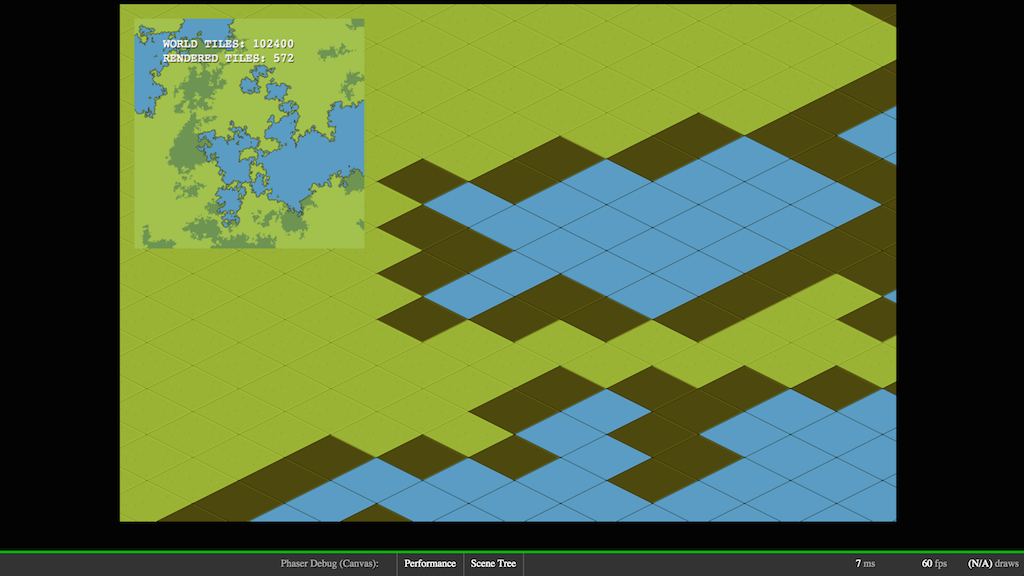## Recommended Posts

Hey all,

I have a giant world and have created a small minimap pixel-by-pixel using ctx.createImageData()

I was wondering if I can take this imagedata and create a sprite from it, so I can use phaser's scale, click events, and other neat features?`        createImage: function() {            var c   = this.game.canvas;            var ctx = c.getContext("2d");            var imgData = ctx.createImageData( _mapGrid.w, _mapGrid.h);                        var imgindex = 0; // increase by 4                                    _mapGrid.each(function(g) {                var pixel = _mapColors[ g.get().type ];                                imgData.data[imgindex+0]=pixel;                imgData.data[imgindex+1]=pixel;                imgData.data[imgindex+2]=pixel;                imgData.data[imgindex+3]=255;                                imgindex += 4;            });                        this.minimap = imgData;        },`
##### Share on other sites

Ended up browsing the docs and noticed game.add.sprite can take a "BitmapData" object, so I looked at Phaser.BitmapData and figured it out

`        createImage: function() {            var bmd = this.game.make.bitmapData( _mapGrid.w, _mapGrid.h );                        var i = 0;            var flatmap = _mapGrid.getAllObj();            bmd.processPixelRGB(function(pixel) {                var tile = flatmap[i];                var color = _mapColors[ tile.type ];                                pixel.r = color;                pixel.g = color;                pixel.b = color;                pixel.a = 255;                                i++;                return pixel;            }, this);         }`

( which then I just added to this.minimap = game.add.sprite(x,y, bmd); )